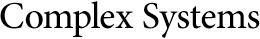## A Cellular Automaton for Burgers' Equation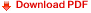Bruce M. Boghosian
Thinking Machines Corporation,
245 First Street, Cambridge, MA 02142-1214, USA

C. David Levermore
Lawrence Levermore National Laboratory,
University of California
Levermore, CA 94550, USA

#### Abstract

We study the approximation of solutions to the Burgers' equation,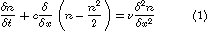by spatially averaging a probabilistic cellular automaton motivated by random walks on a line. The automaton consists of moving "particles" on a one-dimensional periodic lattice with speed one and in a random direction subject to the exclusion principle that at most one particle may move in a given direction from a given lattice site, at a given time. The exclusion principle gives rise to the nonlinearity in Eq. (1) and introduces correlations between the particles which must be estimated to obtain statistical bounds on the error. These bounds are obtained in two steps. The first is showing that the ensemble average of the automaton is a stable explicit finite differencing scheme of Eq. (1) over the lattice with a second order convergence in the lattice spacing. The numerical diffusion of this scheme plays an important role in relating the automaton to Eq. (1). The next step is showing that the spatial averaging of a single evolution of the automaton converges to the spatial averaging of the ensembles as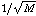where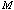is the number of lattice sites averaged. Simulations are presented and discussed.